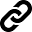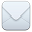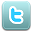SHARE:# Hierarchical Bayes estimation of small area proportions using statistical linkage of disparate data sources

#### General Information

Title
Hierarchical Bayes estimation of small area proportions using statistical linkage of disparate data sources
Author
Soumojit Das, Partha Lahiri
Publication Type
Journal paper
Outlet
Year
2022
Abstract
We propose a Bayesian approach to estimate finite population proportions for small areas. The proposed methodology improves on the traditional sample survey methods because, unlike the traditional methods, our proposed method borrows strength from multiple data sources. Our approach is fundamentally different from the existing small area Bayesian approach to the finite population sampling, which typically assumes a hierarchical model for all units of the finite population. We assume such model only for the units of the finite population in which the outcome variable observed; because for these units, the assumed model can be checked using existing statistical tools. Modeling unobserved units of the finite population is challenging because the assumed model cannot be checked in the absence of data on the outcome variable. To make reasonable modeling assumptions, we propose to form numerous cells for each small area using factors that potentially influence the binary outcome variable of interest. This strategy is expected to bring some degree of homogeneity within a given cell and also among cells from different small areas that are constructed with the same factor level combination. Instead of modeling true probabilities for unobserved individual units, we assume that population means of cells with the same combination of factor levels are identical across small areas and the population mean of true probabilities for a cell is identical to the mean of true values for the observed units in that cell. We apply our proposed methodology to a real-life COVID-19 survey, linking information from multiple disparate data sources to estimate vaccine-hesitancy rates (proportions) for 50 US states and Washington, D.C. (small areas). We also provide practical ways of model selection that can be applied to a wider class of models under similar setting but for a diverse range of scientific problems.